# Equation of State: Ideal Gas

(diff) ← Older revision | Latest revision (diff) | Newer revision → (diff)

The Ideal Gas Equation of State is given by:

•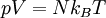$\left. p V = N k_B T \right.$
•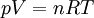$\left. p V = n R T \right.$

where:

•$p$ is the pressure
•$V$ is the volume
•$N$ is the number of molecules
•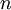$n$ is the number of moles
•$T$ is the absolute temperature
•$k_B$ is the Boltzmann's constant
•$R$ is the Gas constant;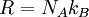$R = N_A k_B$, with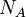$N_A$ being Avogadro constant